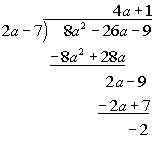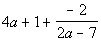# Intermediate Algebra Tutorial 36

Intermediate Algebra
Tutorial 36: Practice Test on Tutorials 32 - 35

Problem 1a: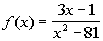Answer:

Our restriction here is that the denominator of a fraction can never be equal to 0.  So, to find our domain, we want to set the denominator "not equal" to 0 to restrict those values.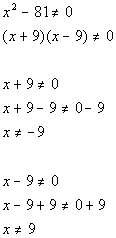Our domain is all real numbers except -9 and 9, because they both make the denominator equal to 0, which would not give us a real number answer for our function.

Problem 2a: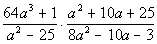Answer: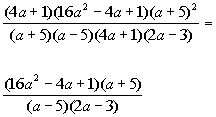Problem 2b: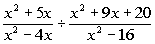Answer: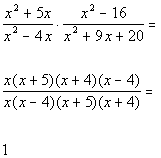Problem 2c: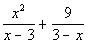Answer:

Step 1: Find the LCD if needed.

The first denominator has the following factor: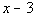The second denominator, 3 - x, looks like the first denominator except the  signs are switched.  We can rewrite this as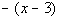Putting all the different factors together and using the highest exponent, we get the following LCD: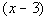Step 2: Write equivalent fractions using the LCD if needed.

Now the two fractions have a common denominator, so we do not have to  rewrite the rational expressions.

Step 3: Combine the rational expressions

AND

Step 4: Reduce to lowest terms.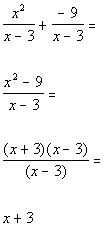Problem 2d: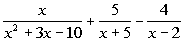Answer:

Step 1: Find the LCD if needed.

The first denominator has the following two factors: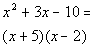The second denominator has the following factor: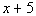The third denominator has the following factor: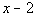Putting all the different factors together and using the highest exponent, we get the following LCD: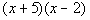Step 2: Write equivalent fractions using the LCD if needed.

Since the first rational expression already has the LCD, then we do not need to change this fraction.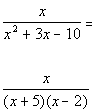Rewriting the second expression with the LCD: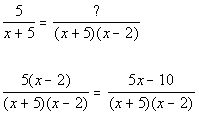Rewriting the third expression with the LCD: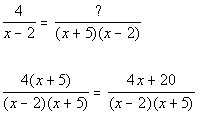Step 3: Combine the rational expressions

AND

Step 4: Reduce to lowest terms.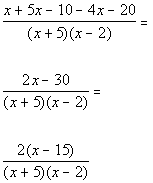Problem 3a: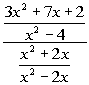Answer:

Note that I used method I described in Tutorial 34 (Complex Fractions) to work this problem.  It is perfectly find to use method II here.

Step 1:   If needed, rewrite the numerator and denominator so  that they are each a single fraction

AND

Step 2:  Divide the numerator by the denominator by multiplying  the numerator by the reciprocal of the denominator

AND

Step 3: If needed, simplify the rational expression.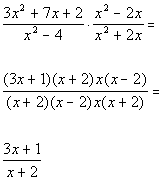Problem 3b: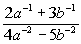Answer:

Note that I used method II described in Tutorial 34: Complex Fractions to work this problem.  It is perfectly fine to use method I here.

Rewriting it with positive exponents we get: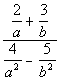Step 1: Multiply the numerator and denominator of the overall complex fractions by the LCD of the smaller fractions

AND

Step 2: If needed, simplify the rational expression.

The two denominators of the numerator's fractions have the  following factors:    a and b

The two denominators of the denominator's fractions  have the  following factors: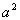and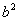Putting all the different factors together and using the highest exponent we get the following LCD for all the small fractions: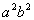Multiplying numerator and denominator by the LCD and simplifying we get: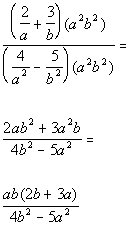Problem 4a: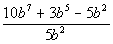Answer: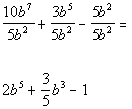Problem 4b: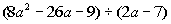Answer:

Step 1: Set up the long division

AND

Step 2: Divide 1st term of divisor by first term of dividend to get first term of the quotient

AND

Step 3:  Take the term found in step 1 and multiply it times the divisor

AND

Step 4:  Subtract this from the line above

AND

Step 5:  Repeat until done

AND

Step 6: Write out the answer.